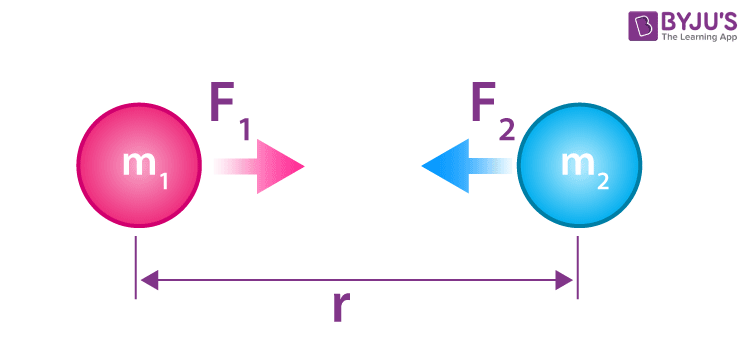What is the formula for Newton's law of universal gravitation?

Newton’s Law of Universal Gravitation states that every particle attracts every other particle in the universe with force directly proportional to the product of the masses and inversely proportional to the square of the distance between them.

Definition

Newton’s Law of Universal Gravitation states that every particle attracts every other particle in the universe with force directly proportional to the product of the masses and inversely proportional to the square of the distance between them.

Universal Gravitation Equation$$F\propto \frac{m_{1}m_{2}}{r^2}$$ $$F= G\frac{m_{1}m_{2}}{r^2}$$

Where,

• F is the gravitational force between bodies
• m1 and m2 are the masses of the bodies
• r is the distance between the centres of two bodies
• G is the universal gravitational constant (6.673 x 10-11 N m2/kg2)
•Articles to Explore:(3)(0)